Next: Discussion Up: No Title Previous: Parameters of binary compact

# Detection rate of binary compact star merging

Under the assumptions made above, we can calculate the binary merging rates in the Galaxy R. The results are presented in Fig.and.Figure: The dependence of different compact binary merging rates in a model ``elliptical'' galaxy (where all the stars were formed simultaneously at the moment t=0) with massM. The horizontal line shows the mean merging rate of binary NS in a spiral galaxy of the same mass with a stationary star formation.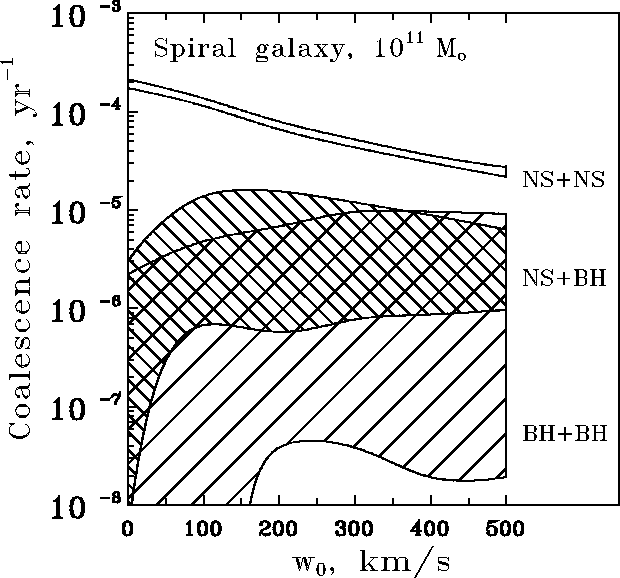Figure: The dependence of different compact binary merging rates in a spiral galaxy withMon the characteristic kick velocity.

After having found the merging rates R in a typical galaxy, we need to go over the event rate D at the detector. Applying the optimal filtering technique , the signal-to-noise ratio S/N at the spiral-in stage isHereis ``chorp''-mass of the binary system. This means that for a given S/N our detector can register more massive BH from larger distances than NS. The volume within which BH or NS is to be detected should be proportional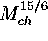. Then the ratio of detection rates of BH and NS can be written as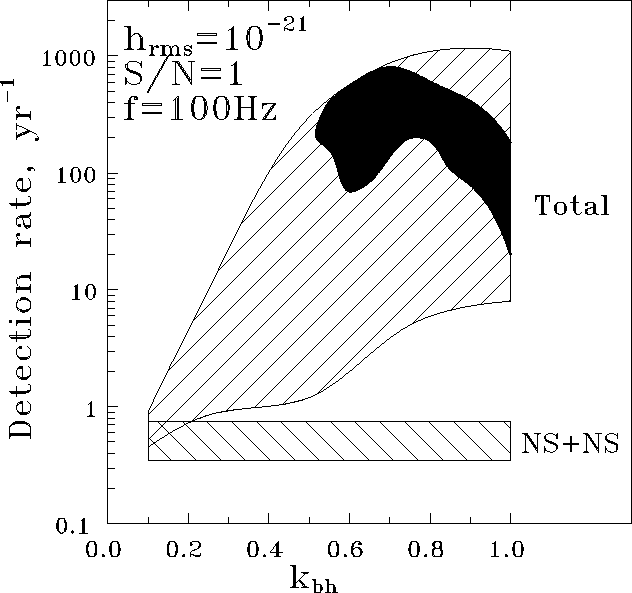Figure: The total merging rate of NS+NS, NS+BH, and BH+BH binaries as would be detected by a laser interferometer with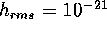for Lyne-Lorimer kick velocity distribution with-400 km/s and BH progenitor's masses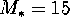-50M, for different scenarios of binary star evolution as a function of. NS+NS mergings are shown separately. In all cases BH+BH mergings contribute more thanto the total rate. The filled ``Loch-Ness-monster-head''-like region corresponds to BH formation parametersMand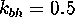.

Let us make a simple estimate. Take a GW detector withand S/N=1 (as for the initial LIGO or VIRGO interferometer). Let the mass of NS and BH be 1.4M(the typical value well justified experimentally) and 8.5M(the mean mass of the BH candidates, see Table). Let us also assume that any star with the initial mass M(NS)>10Myields NS (the typical value confirmed theoretically), and the threshold mass for BH formation is the maximal from the estimates given above,M. Hence using Salpeter mass function we find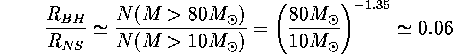and thenThis estimate is, of course, very rough, but the precise calculation gives essentially the same result. Fig.shows the calculated absolute registration rate of mergings D by the detector withand S/N=1, as a function of. The calculations have been done for Lyne-Lorimer kick velocity distribution with the parameter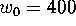ËÍ/Ó. The vertical dispersion is due to the dependence of D on. It is seen that binary NS mergings occur with a rate of about 1 event per year. In the same time, the total detection rate (except for) can exceed that of binary NS mergings by 2-3 orders. The filled region shows the region of the ``most probable parameters'', for which the calculations meet both the upper limit to the number of BH with radiopulsars (less than 1 per 700 single pulsars) and the number of BH candidates of Cyg X-1 type (from 1 to 10 in the Galaxy). In this region, from 10 to 100 mergings per year must be detected, with the majority of them being binary BH mergings.Next: Discussion Up: No Title Previous: Parameters of binary compact

Lipunov V.M.
Fri Nov 28 17:12:56 MSK 1997